# Easy To Use Math Help: Fraction Help Mats

There are some math concepts that are always hard to learn. Fractions can be one of them. There are so many different ways to look and use fractions.

I wanted to create something that would help kids learn their fractions and help with the struggle at the start. I made these fraction help mats.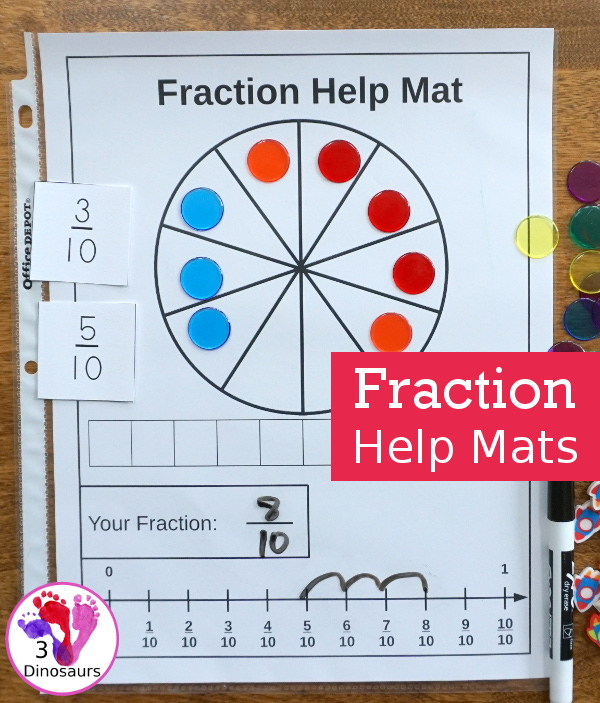These mats are a great way to look at the number line of the fraction and see how it works in different layouts. I love using hands-on activities when I can.

Each of the mats has a fraction at the top, a horizontal fraction, a number line and recording area. It gives kids loads of ways to work on the fractions.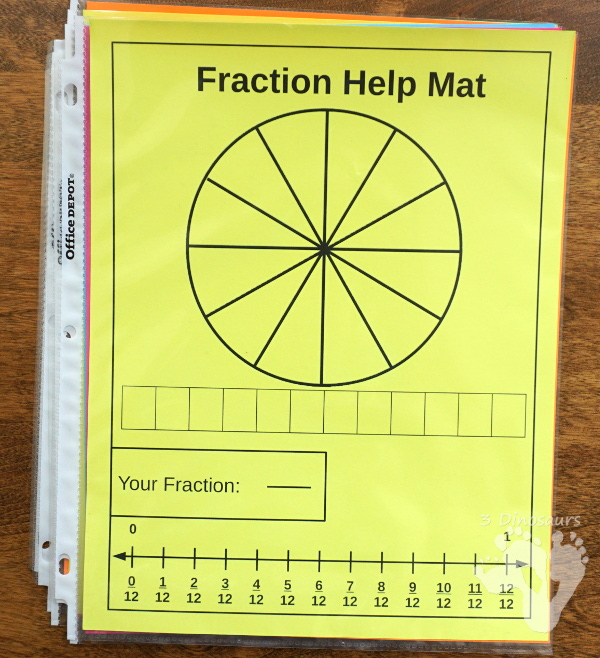There are 8 mats in all. I have printed out off on colored card stock. Then I put them in sheet protectors back to back. This allows me to put them in our math help binder. The mats have the following fractions: 12, 10, 8, 6, 5, 4, 3, and 2.

You can see the maths below. I printed them on colored cardstock and put them in sheet protects back to back. We store these in our math help binder.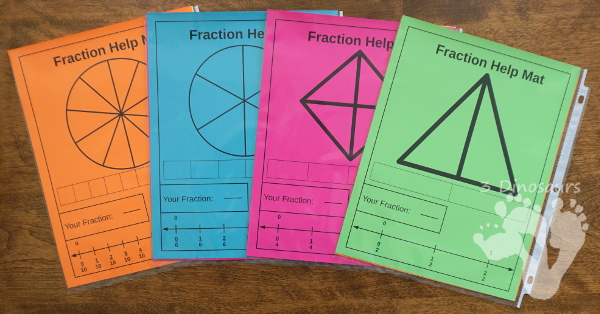## How we used these mats

We have used these mats with some of the printables on the site. The most recent has been the fraction matching. The cards have repeat addition on them so it makes it great to see you build the fraction and match it on the number line. You can see how we used the card on the number line to add the fractions up. Then we filled out the visual fractions with erasers to make the equation.You can use the mats to add two fractions together that have the same denominator. You can see we took two fractions cards and added them together. We got the fraction cards from the Comparing Stormtrooper Fractions printable.You can also use these cards to figure out fractions. You can count the spots and get the matching mat. Then use the number line to see what the fraction is.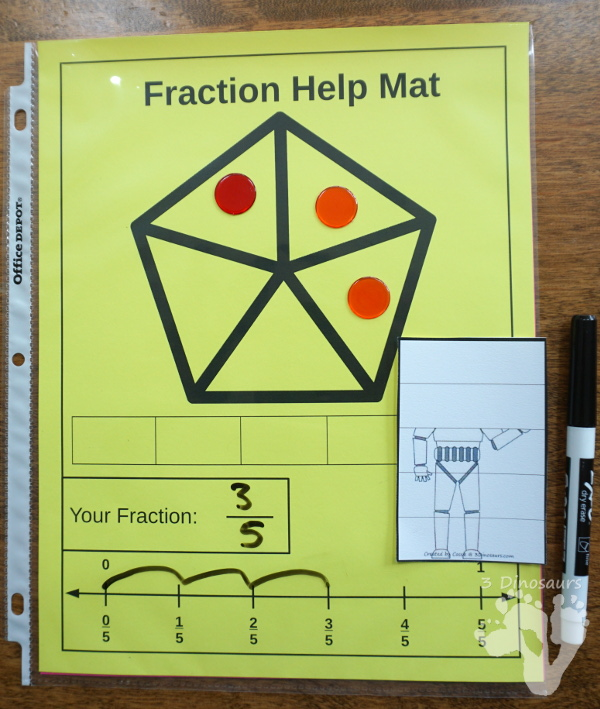## What you will find in the free Fraction Help Mats

• 8 Fraction Help Mats
• Fractions for: 12, 10, 8, 6, 5, 4, 3, and 2
• Two fraction examples to color in
• Number line from 0 to 1 with fractions marked off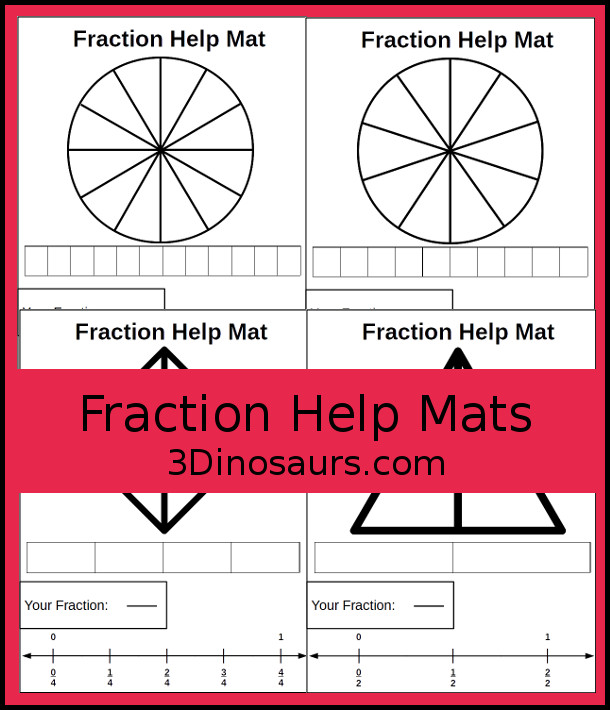Check out these other fraction printables:

Cassie – 3Dinosaurs.com